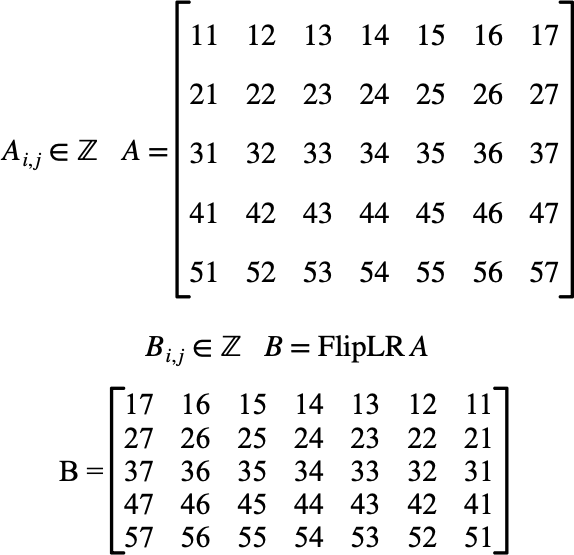# $$\text{FlipLR}$$¶

You can use the $$\text{FlipLR}$$ function to reverse the column order of a matrix.

You can use the \fliplr backslash command to insert this function.

The following variants of this function are available:

• $$\text{matrix } \text{FlipLR} \left ( \text{<matrix>} \right )$$

The $$\text{<matrix>}$$ parameter is any type of matrix. The $$\text{FlipLR}$$ function will return a matrix of the same type and the same dimension with the columns reversed.

The Figure 139 shows how you can use the $$\text{FlipLR}$$ function.Figure 139 Example Use Of The FlipLR Function